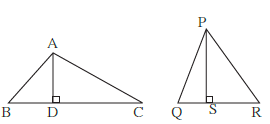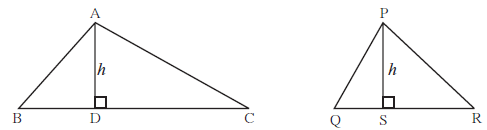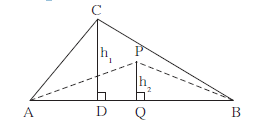# Properties of Ratios of Areas of Two Triangles

#### notes

We have studied Ratio and Proportion. The statement, "the numbers a and b are in the ratio m/n " is also written as, "the numbers a and b are in proportion m:n". For this concept we consider positive real numbers. We know that the lengths of line segments and area of any figure are positive real numbers. We know the formula of area of a triangle.
Area of a triangle =1/2 Base × Height

Let’s find the ratio of areas of any two triangles.

Ex. In D ABC, AD is the height and BC is the base. In D PQR, PS is the height and QR is the base.

A(triangle ABC)/A(trianglePQR)=(1/2xxBCxxAD)/(1/2xxQRxxPS)A(triangle ABC)/A(triangle PQR)=(BCxxAD)/(QRxxPS)

Hence the ratio of the areas of two triangles is equal to the ratio of the products of their bases and corrosponding heights. Base of a triangle is b1 and height is h1. Base of another triangle is b2 and height is h2 .

Then the ratio of their areas =(b_1xxh_1)/(b_2xxh_2)

Suppose some conditions are imposed on these two triangles,

Condition 1: If the heights of both triangles are equal then :A(triangle ABC)/A(triangle PQR)=(BCxxh)/(QRxxh)="BC"/QR"

A(triangle ABC)/A(triangle PQR)=b_1/b_2

Property: The ratio of the areas of two triangles with equal heights is equal to the ratio of their corresponding bases.

Condition 2: If the bases of both triangles are equal then -A(triangle ABC)/A(triangle APB)=(ABxxh_1)/(ABxxh_2)

A(triangle ABC)/A(triangle APB)=h_1/h_2

Property: The ratio of the areas of two triangles with equal bases is equal to the ratio of their corresponding heights.

If you would like to contribute notes or other learning material, please submit them using the button below.

### Shaalaa.com

Similarity Ex 1.1 Properties of ratios of areas of two triangles [00:19:32]
S
0%